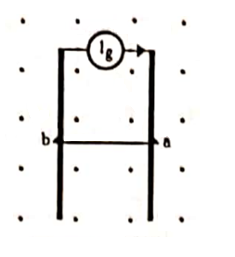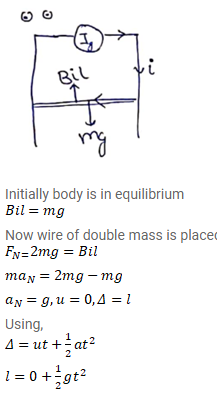# The system containing the rails and the wire of the previous problem is kept vertically

Question:

The system containing the rails and the wire of the previous problem is kept vertically in a uniform horizontal magnetic field $\mathrm{B}$ that is perpendicular to the rails. It is found that the wire stays in equilibrium. If the wire ab is replaced by another wire of double its mass, how long will it take in falling through a distance equal to its length?Solution:$t=\sqrt{\frac{2 l}{g}}$